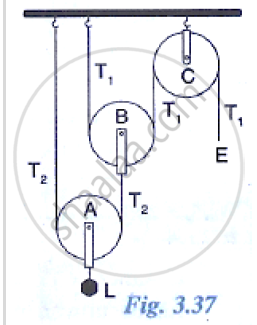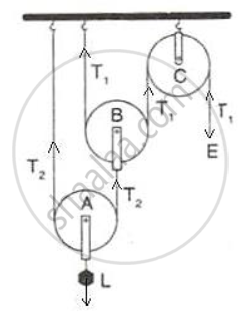Share
Notifications

View all notifications
Books Shortlist
Your shortlist is empty

# The Diagram Alongside Shows an Arrangement of Three Pulleys Name the Pulleys A, B, and C.Mark in the Diagram the Directions of Load (L), Effort (E) and Tension T1 and T2 in the Two Strings. - ICSE Class 10 - Physics

Login
Create free account

Forgot password?

#### Question

The Diagram alongside shows an arrangement of three pulleys A, B, and C. The load is marked as L and the effort as E.
(a) Name the Pulleys A, B, and C.
(b) Mark in the diagram the directions of load (L), effort (E) and tension T1 and T2 in the two strings.
(c) How are the magnitudes of L and E related to the tension T1?
(d) Calculate the mechanical advantage and velocity ratio of the arrangement.
(e) What assumptions have you made in parts (c) and (d)?#### Solution

(a) Pulleys A and B are movable pulleys. Pulley C is fixed pulley.
(b)(c) The magnitude of effort E = T1
And the magnitude of L= 22 T1 = 4 T1
(d) The mechanical advantage = 22 = 4
The velocity ratio = 22 = 4
(e) Assumption: the pulleys A and B are weightless.

Is there an error in this question or solution?

#### APPEARS IN

Solution The Diagram Alongside Shows an Arrangement of Three Pulleys Name the Pulleys A, B, and C.Mark in the Diagram the Directions of Load (L), Effort (E) and Tension T1 and T2 in the Two Strings. Concept: Concept of Pulley System.
S